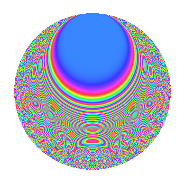# Properties

 Label 6017.2.a.fLevel 6017 Weight 2 Character orbit 6017.a Self dual Yes Analytic conductor 48.046 Analytic rank 0 Dimension 121 CM No

# Related objects

## Newspace parameters

 Level: $$N$$ = $$6017 = 11 \cdot 547$$ Weight: $$k$$ = $$2$$ Character orbit: $$[\chi]$$ = 6017.a (trivial)

## Newform invariants

 Self dual: Yes Analytic conductor: $$48.0459868962$$ Analytic rank: $$0$$ Dimension: $$121$$ Fricke sign: $$-1$$ Sato-Tate group: $\mathrm{SU}(2)$

## $q$-expansion

The dimension is sufficiently large that we do not compute an algebraic $$q$$-expansion, but we have computed the trace expansion.

 $$\operatorname{Tr}(f)(q) =$$ $$121q$$ $$\mathstrut +\mathstrut 2q^{2}$$ $$\mathstrut +\mathstrut 18q^{3}$$ $$\mathstrut +\mathstrut 138q^{4}$$ $$\mathstrut +\mathstrut 13q^{5}$$ $$\mathstrut +\mathstrut 10q^{6}$$ $$\mathstrut +\mathstrut 56q^{7}$$ $$\mathstrut +\mathstrut 12q^{8}$$ $$\mathstrut +\mathstrut 143q^{9}$$ $$\mathstrut +\mathstrut O(q^{10})$$ $$\operatorname{Tr}(f)(q) =$$ $$121q$$ $$\mathstrut +\mathstrut 2q^{2}$$ $$\mathstrut +\mathstrut 18q^{3}$$ $$\mathstrut +\mathstrut 138q^{4}$$ $$\mathstrut +\mathstrut 13q^{5}$$ $$\mathstrut +\mathstrut 10q^{6}$$ $$\mathstrut +\mathstrut 56q^{7}$$ $$\mathstrut +\mathstrut 12q^{8}$$ $$\mathstrut +\mathstrut 143q^{9}$$ $$\mathstrut +\mathstrut 20q^{10}$$ $$\mathstrut -\mathstrut 121q^{11}$$ $$\mathstrut +\mathstrut 40q^{12}$$ $$\mathstrut +\mathstrut 31q^{13}$$ $$\mathstrut +\mathstrut 7q^{14}$$ $$\mathstrut +\mathstrut 53q^{15}$$ $$\mathstrut +\mathstrut 164q^{16}$$ $$\mathstrut -\mathstrut 23q^{17}$$ $$\mathstrut +\mathstrut 14q^{18}$$ $$\mathstrut +\mathstrut 62q^{19}$$ $$\mathstrut +\mathstrut 53q^{20}$$ $$\mathstrut +\mathstrut 19q^{21}$$ $$\mathstrut -\mathstrut 2q^{22}$$ $$\mathstrut +\mathstrut 34q^{23}$$ $$\mathstrut +\mathstrut 34q^{24}$$ $$\mathstrut +\mathstrut 172q^{25}$$ $$\mathstrut +\mathstrut 34q^{26}$$ $$\mathstrut +\mathstrut 87q^{27}$$ $$\mathstrut +\mathstrut 91q^{28}$$ $$\mathstrut -\mathstrut 30q^{29}$$ $$\mathstrut +\mathstrut 2q^{30}$$ $$\mathstrut +\mathstrut 102q^{31}$$ $$\mathstrut +\mathstrut 31q^{32}$$ $$\mathstrut -\mathstrut 18q^{33}$$ $$\mathstrut +\mathstrut 30q^{34}$$ $$\mathstrut +\mathstrut 20q^{35}$$ $$\mathstrut +\mathstrut 164q^{36}$$ $$\mathstrut +\mathstrut 58q^{37}$$ $$\mathstrut +\mathstrut 35q^{38}$$ $$\mathstrut +\mathstrut 42q^{39}$$ $$\mathstrut +\mathstrut 52q^{40}$$ $$\mathstrut -\mathstrut 12q^{41}$$ $$\mathstrut +\mathstrut 56q^{42}$$ $$\mathstrut +\mathstrut 96q^{43}$$ $$\mathstrut -\mathstrut 138q^{44}$$ $$\mathstrut +\mathstrut 72q^{45}$$ $$\mathstrut +\mathstrut 48q^{46}$$ $$\mathstrut +\mathstrut 136q^{47}$$ $$\mathstrut +\mathstrut 99q^{48}$$ $$\mathstrut +\mathstrut 199q^{49}$$ $$\mathstrut -\mathstrut 7q^{50}$$ $$\mathstrut +\mathstrut 22q^{51}$$ $$\mathstrut +\mathstrut 81q^{52}$$ $$\mathstrut +\mathstrut 24q^{53}$$ $$\mathstrut +\mathstrut 37q^{54}$$ $$\mathstrut -\mathstrut 13q^{55}$$ $$\mathstrut +\mathstrut 28q^{56}$$ $$\mathstrut +\mathstrut 25q^{57}$$ $$\mathstrut +\mathstrut 76q^{58}$$ $$\mathstrut +\mathstrut 58q^{59}$$ $$\mathstrut +\mathstrut 81q^{60}$$ $$\mathstrut +\mathstrut 14q^{61}$$ $$\mathstrut -\mathstrut 2q^{62}$$ $$\mathstrut +\mathstrut 152q^{63}$$ $$\mathstrut +\mathstrut 236q^{64}$$ $$\mathstrut -\mathstrut 29q^{65}$$ $$\mathstrut -\mathstrut 10q^{66}$$ $$\mathstrut +\mathstrut 112q^{67}$$ $$\mathstrut -\mathstrut 61q^{68}$$ $$\mathstrut +\mathstrut 41q^{69}$$ $$\mathstrut +\mathstrut 105q^{70}$$ $$\mathstrut +\mathstrut 56q^{71}$$ $$\mathstrut +\mathstrut 71q^{72}$$ $$\mathstrut +\mathstrut 113q^{73}$$ $$\mathstrut -\mathstrut 23q^{74}$$ $$\mathstrut +\mathstrut 111q^{75}$$ $$\mathstrut +\mathstrut 144q^{76}$$ $$\mathstrut -\mathstrut 56q^{77}$$ $$\mathstrut +\mathstrut 59q^{78}$$ $$\mathstrut +\mathstrut 80q^{79}$$ $$\mathstrut +\mathstrut 100q^{80}$$ $$\mathstrut +\mathstrut 177q^{81}$$ $$\mathstrut +\mathstrut 123q^{82}$$ $$\mathstrut +\mathstrut 6q^{83}$$ $$\mathstrut +\mathstrut 79q^{84}$$ $$\mathstrut +\mathstrut 26q^{85}$$ $$\mathstrut +\mathstrut 14q^{86}$$ $$\mathstrut +\mathstrut 180q^{87}$$ $$\mathstrut -\mathstrut 12q^{88}$$ $$\mathstrut +\mathstrut 26q^{89}$$ $$\mathstrut +\mathstrut 75q^{90}$$ $$\mathstrut +\mathstrut 72q^{91}$$ $$\mathstrut +\mathstrut 58q^{92}$$ $$\mathstrut +\mathstrut 139q^{93}$$ $$\mathstrut +\mathstrut 37q^{94}$$ $$\mathstrut +\mathstrut 39q^{95}$$ $$\mathstrut +\mathstrut 66q^{96}$$ $$\mathstrut +\mathstrut 136q^{97}$$ $$\mathstrut +\mathstrut 7q^{98}$$ $$\mathstrut -\mathstrut 143q^{99}$$ $$\mathstrut +\mathstrut O(q^{100})$$

## Embeddings

For each embedding $$\iota_m$$ of the coefficient field, the values $$\iota_m(a_n)$$ are shown below.

For more information on an embedded modular form you can click on its label.

Label $$a_{2}$$ $$a_{3}$$ $$a_{4}$$ $$a_{5}$$ $$a_{6}$$ $$a_{7}$$ $$a_{8}$$ $$a_{9}$$ $$a_{10}$$
1.1 −2.76609 1.72204 5.65124 1.59310 −4.76331 4.82706 −10.0996 −0.0345853 −4.40666
1.2 −2.74533 −1.76027 5.53683 −2.91942 4.83253 1.39362 −9.70977 0.0985659 8.01478
1.3 −2.73908 1.22981 5.50258 −1.73351 −3.36855 −3.95971 −9.59385 −1.48757 4.74824
1.4 −2.69280 3.13292 5.25118 0.629801 −8.43634 −1.84618 −8.75479 6.81521 −1.69593
1.5 −2.68922 1.49908 5.23192 −2.86648 −4.03136 3.78429 −8.69136 −0.752759 7.70861
1.6 −2.66480 −1.17356 5.10118 4.42559 3.12731 1.20677 −8.26404 −1.62275 −11.7933
1.7 −2.61029 −0.993650 4.81359 −3.58363 2.59371 2.54427 −7.34428 −2.01266 9.35430
1.8 −2.58820 0.659368 4.69880 0.894285 −1.70658 −1.01597 −6.98504 −2.56523 −2.31459
1.9 −2.57735 −3.20978 4.64274 2.33292 8.27272 1.38140 −6.81126 7.30267 −6.01276
1.10 −2.54623 −1.76925 4.48328 −0.663879 4.50492 −3.59601 −6.32301 0.130248 1.69039
1.11 −2.47328 −1.34586 4.11710 2.94670 3.32869 −2.85130 −5.23618 −1.18866 −7.28801
1.12 −2.37440 −0.379878 3.63775 −1.52566 0.901981 2.31296 −3.88867 −2.85569 3.62251
1.13 −2.32206 0.535084 3.39196 −0.511363 −1.24250 3.83500 −3.23221 −2.71368 1.18742
1.14 −2.30378 2.53807 3.30742 3.98214 −5.84717 −4.26603 −3.01201 3.44182 −9.17400
1.15 −2.29477 3.40179 3.26597 3.88142 −7.80633 2.49357 −2.90510 8.57220 −8.90697
1.16 −2.27604 3.24984 3.18035 −3.77187 −7.39676 3.04189 −2.68652 7.56146 8.58492
1.17 −2.26471 2.40007 3.12892 2.90605 −5.43547 2.22739 −2.55669 2.76034 −6.58136
1.18 −2.25579 −2.77556 3.08857 0.288075 6.26106 1.78637 −2.45557 4.70373 −0.649836
1.19 −2.22670 0.200688 2.95817 3.39958 −0.446870 −0.867591 −2.13356 −2.95972 −7.56983
1.20 −2.10704 −1.55624 2.43961 −0.464512 3.27907 −0.781558 −0.926282 −0.578102 0.978744
See next 80 embeddings (of 121 total)
 $$n$$: e.g. 2-40 or 990-1000 Embeddings: e.g. 1-3 or 1.121 Significant digits: Format: Complex embeddings Normalized embeddings Satake parameters Satake angles

## Inner twists

This newform does not have CM; other inner twists have not been computed.

## Atkin-Lehner signs

$$p$$ Sign
$$11$$ $$1$$
$$547$$ $$-1$$

## Hecke kernels

This newform can be constructed as the intersection of the kernels of the following linear operators acting on $$S_{2}^{\mathrm{new}}(\Gamma_0(6017))$$:

 $$T_{2}^{121} - \cdots$$ $$T_{3}^{121} - \cdots$$